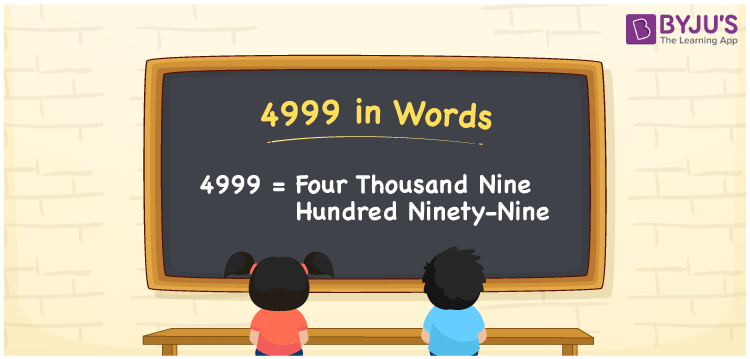# 4999 in Words

We can write 4999 in words as Four thousand nine hundred ninety-nine. In general, we use the word form of numbers to express or communicate the numerical quantity or measure. For example, Rs. 4999 can be communicated as “Four thousand nine hundred ninety-nine rupees”. However, we can get these word forms using a place value chart. In this article, you will get the conversion of the cardinal number 4999 into words.

 4999 in words Four thousand nine hundred ninety-nine Four thousand nine hundred ninety-nine in Numbers 4999

## 4999 in English Words

In general, we express numbers in words using the English alphabet. Thus, we can spell 4999 in English words as “Four thousand nine hundred ninety-nine”.## How to Write 4999 in Words?

Conversion of the number 4999 into word form can be done easily with the help of a place value chart, as shown below.

 Thousands Hundreds Tens Ones 4 9 9 9

Here, ones = 9, tens = 9, hundreds = 9, and thousands = 4.

Thus, we can expand the above digits as per their place values as:

4 × Thousand + 9 × Hundred + 9 × Ten + 9 × One

= 4 × 1000 + 9 × 100 + 9 × 10 + 9 × 1

= 4000 + 900 + 90 + 9

= Four thousand + Nine hundred + Ninety + Nine

= Four thousand nine hundred ninety-nine

Therefore, 4999 in words = Four thousand nine hundred ninety-nine.

As we know, 4999 is a natural number that precedes 5000 and succeeds 4998.

4999 in words – Four thousand nine hundred ninety-nine

Is 4999 an odd number? – Yes

Is 4999 an even number? – No

Is 4999 a composite number? – No

Is 4999 a prime number? – Yes

Is 4999 a perfect square number? – No

Is 4999 a perfect cube number? – No

## Frequently Asked Questions on 4999 in Words

### How do you write 4999 in words?

We can write the number 4999 in words as Four thousand nine hundred ninety-nine.

### How to write Rs. 4999 in words on a cheque?

On a cheque, we can express Rs. 4999 in words as “Four thousand nine hundred ninety-nine rupees only”.

### Express the value of 4999 + 5000 in words.

4999 + 5000 = 9999 Hence, we can express the value of 4999 + 5000, i.e., 9999 in words as Nine thousand nine hundred ninety-nine.